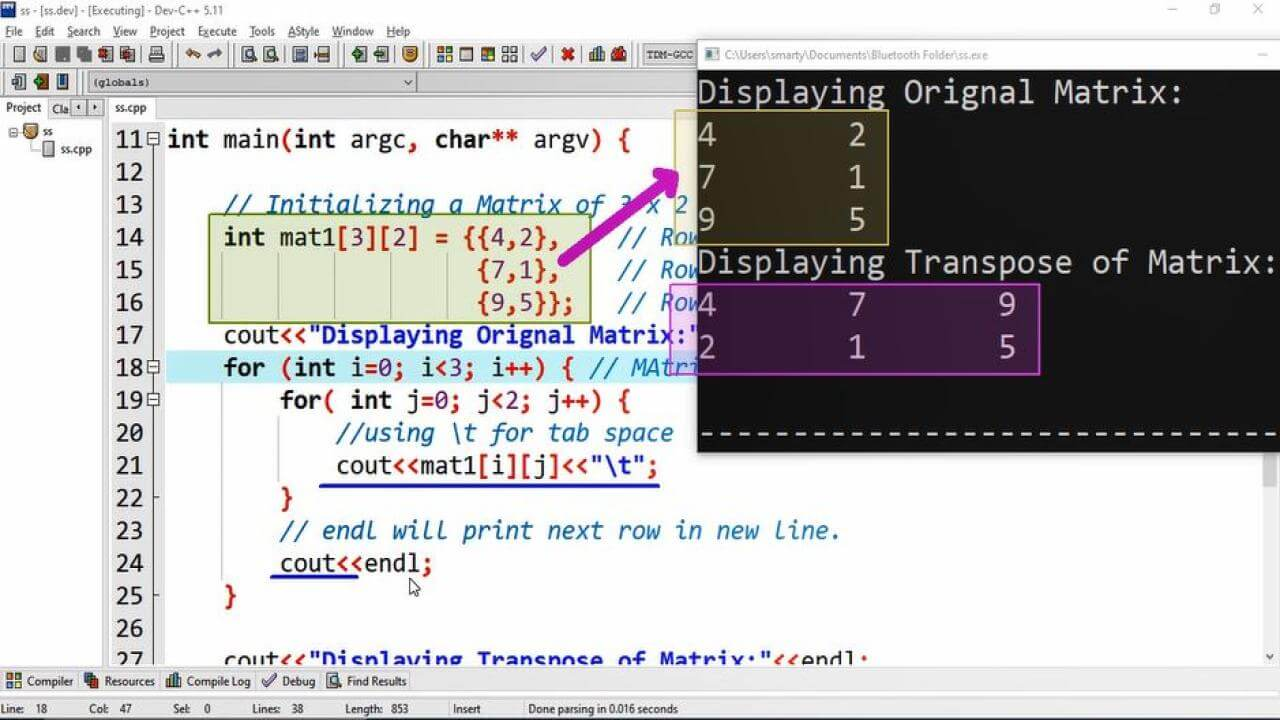# Matrix Transpose Program Example using CPP

## Source Code:

#include <iostream>
using namespace std;

/*
Matrix A = 3 x 2 Then
Transpose of Matrix A = 2 x 3
Rows will be Columns in Resultant
Matrix.
*/

int main(int argc, char** argv) {

// Initializing a Matrix of 3 x 2
int mat1 = {{4,2},    // Row 1
{7,1},    // Row 2
{9,5}};    // Row 3
cout<<"Displaying Orignal Matrix:"<<endl;
for (int i=0; i<3; i++) { // MAtrix in MxN order
for( int j=0; j<2; j++) {
//using \t for tab space
cout<<mat1[i][j]<<"\t";
}
// endl will print next row in new line.
cout<<endl;
}

cout<<"Displaying Transpose of Matrix:"<<endl;
for (int i=0; i<2; i++) { // MAtrix in NxM order
for( int j=0; j<3; j++) {
//using \t for tab space
cout<<mat1[j][i]<<"\t";
}
// endl will print next row in new line.
cout<<endl;
}

return 0;
}

## Output:## Working:

In this program example we are going to get Matrix Transpose using nested while loop. For this purpose we have declared an array of order 3 x 2 and initialize it with some random integer values. After initialization proess we use nested loop to print this orignal matrix.

Whenever we declare a two dimentional array, its first index represent its rows and second represent its columns.

To get transpose we do not use any other temporary array of perform any other kind of calculations. We just simply replace the condition of inner and outer loop. So that this nested loop will print rows as columns and columns as rows. After  printing each value of matrix we print some tab space using \t escape sequence. The purpose of using endl is that we are going to print every next row of matrix in new line.